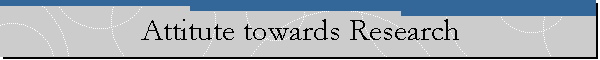My general attitude towards research is best described by saying that I chase patterns. When I see a good result I want to know what is happening behind the scenes that causes this result and once I find the facts that caused the beautiful result I indicate how else those facts can be used. Most of my papers are good examples of that but my work on Nagata like theorems and on Kaplansky like theorems stands out. Here Nagata like Theorems are statements that generalize Nagata's theorem on UFD's: Let R be an integral domain that satisfies ACC on principal ideals, and let S be a multiplicative set generated by primes of R,. If is a UFD then so is R. Next, the theorem of Kaplansky that I targeted is: An integral domain R is a UFD if and only if, every nonzero prime ideal of R contains a nonzero principal prime. I have singled out these two theorems because I was so impressed by them that I actually went chasing their patterns into partially ordered groups (not necessarily abelian) and came up with some interesting results.

back